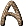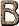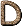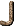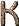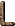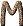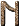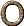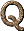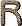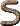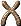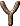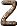G Spectral type for yellow stars, such as the Sun, Alpha Centauri A, and Capella. [C95] G\$ Giga US Dollars. [LLM96] G-band A band of CH at 4303 Å. It is conspicuous in the spectra of G-K stars. [H76] g-factor Ratio of a particle's magnetic moment to its spin angular momentum. [H76] gf-values Weighted oscillator strengths. f = oscillator strength of the transition: g = statistical weight of the lower level. [H76] G star Stars of spectral type G are yellowish stars with surface temperatures of about 5000 to 6000 K, in which the H and K lines of Ca II have become dominant and in which a tremendous profusion of spectral lines of both neutral and ionized metals, particularly iron, begins to show. The Balmer lines of hydrogen are still recognizable. Examples are the Sun and Capella. [H76] GadoliniumA ductile malleable silvery element of the lanthanoid series of metals. It occurs in association with other lanthanoids. Gadolinium is used in alloys, magnets, and in the electronics industry. Symbol: Gd; m.p. 1313°C; b.p. 3266°C; r.d. 7.9 (25°C); p.n. 64; r.a.m. 157.25. [DC99] Gain The amplification factor. [McL97] Galactic 1. When capitalized, the word refers to our Galaxy. 2. When not capitalized, it refers to a galaxy. [C95] Galactic Anticenter The point in the Galactic plane that lies directly opposite the Galactic center. Here we gaze toward the edge of the Galactic disk. The nearest bright star to the anticenter is El Nath, in the constellation Taurus. [C95] Galactic Astronomy The study of the Milky Way. [C95] Galactic Centers Are now thought to comprise black holes - which would explain why the centre of our Galaxy appears strangely obscure, and emits only infrared radiation. [A84] Galactic Cluster See open cluster. [H76] Galactic Coordinates A system of coordinates based on the mean plane of the Galaxy, which is inclined about 63° to the celestial equator. Galactic latitude (b) is measured from the galactic equator north (+) or south (-); galactic longitude (l) is measured eastward along the galactic plane from the galactic center. In 1958, because of increased precision in determining the location of the galactic center, a new system of galactic coordinates was adopted, with the origin at the galactic center in Sagittarius at(1950) = 17h42m.4,(1950) = - 28°55'. The new system is designated by a superior roman numeral II (i.e., bII, lII) and the old system by a superior roman numeral I: lIIlI + 32°.31. Galactic coordinates are independent of precession. [H76] Galactic DiskThe plate-shaped component of a spiral galaxy, in which the spiral arms are found. [F88] Galactic Equator The primary circle defined by the central plane of the Galaxy. [H76] Galactic Halo (a) A spherical aggregation of stars, globular star clusters, and thin gas clouds, centered on the nucleus of the galaxy and extending beyond the known extremities of the galactic disk. [F88] (b) The unseen mass that is believed to surround each galaxy and whose gravitational effects are believed to hold the galaxy together. [LB90] Galactic Latitude The angle between the line of sight to a star and the Galactic plane. Galactic latitude ranges from +90 degrees to -90 degrees; the Galactic plane has a Galactic latitude of 0 degrees. Regions north of the Galactic plane have positive Galactic latitude; regions south have negative Galactic latitude. The point with a Galactic latitude of +90 degrees is called the north Galactic pole, and the point with a Galactic latitude of -90 degrees is called the south Galactic pole. [C95] Galactic Light See diffuse galactic light. [H76] Galactic Longitude A measure of a star's position with respect to the Sun and Galactic center. Galactic longitude ranges from 0 degrees to 360 degrees. Imagine the Sun at the center of a giant clock, with the Galactic center located in the direction of six o'clock. A Galactic longitude of 0 degrees would correspond to the direction of six o'clock, a Galactic longitude of 90 degrees to the direction of three o'clock, a Galactic longitude of 180 degrees to the direction of twelve o'clock, and a Galactic longitude of 270 degrees to the direction of nine o'clock. [C95] Galactic NucleusIn the innermost region of a galaxy, there is often a concentration of stars and gas, sometimes extending over thousands of light-years from the center of the galaxy. [Silk90] Galactic Plane The plane that contains the disk of the Milky Way. By definition, one direction perpendicular to this plane is called "above" or "north", and the opposite direction, also perpendicular to the Galactic plane, is called "below" or "south". From Earth, due Galactic north is marked by the north Galactic pole, which lies near the bright star Arcturus, and due Galactic south is marked by the south Galactic pole, which lies in the faint constellation Sculptor. [C95] Galactic Pole (a) Either of the two points in the sky where we look perpendicular to the disk of the Milky Way. The north Galactic pole is the Galactic pole located above the disk; the south Galactic pole is the Galactic pole located below the disk. [C95] (b) The pole of the galactic plane. The "new" system puts the galactic north pole in Coma at(1950) = 12h49m,(1950) = 27°.4. [H76] Galactic Rotation The revolving of a galaxy round its central nucleus even as it continues its proper motion. Such rotation, however, is not uniform but differential. One revolution of the Sun within our own Galaxy takes about 225 million years, or 1 cosmic year. [A84] Galactic Tide See tide. [C95] Galactic Wind A hypothetical outflow of tenuous material from a galaxy, analogous to the solar wind. [H76] Galactocentric Distance A star's distance from the Galactic center. The Sun's Galactocentric distance is about 27,000 light-years. [C95] Galaxy (a) Vast system of celestial objects, typically consisting of between 106 and 1012 stars, plus interstellar gas and dust. There are three basic types: spiral (further subdivided into normal spirals and spirals with a "bar" at the centre, and yet further subdivided according to the "openness" of the spiral arms), elliptical (subdivided according to ellipticity) and irregular (subdivided according to whether they are made up of Population I or Population II stars). Another not uncommon type of galaxy is a lenticular form mid-way between the spiral and the elliptical. [A84] (b) A huge collection of millions, billions, or trillions of stars. When referring to the Milky Way, "galaxy" is capitalized, otherwise not; thus: "Andromeda is the nearest giant galaxy to the Galaxy". [C95] (c) A large aggregation of stars, bound together gravitationally. There are three major classifications of galaxies - spiral, elliptical, and irregular - and several subclassifications. The sun belongs to a spiral galaxy, the Milky Way galaxy. [F88] (d) A large (108-1013 M), gravitationally bound aggregate of stars and interstellar matter. Galaxy formation is currently believed to have occurred around z3-4. [H76] (e) An isolated aggregation of stars and gas, held together by their mutual gravity. A typical galaxy has about 100 billion stars, has a total mass equal to about a trillion times the mass of the sun, is about 100,000 light years in diameter, and is separated from the nearest galaxy by a distance of about 100 times its own diameter. Thus, galaxies are islands of stars in space. Our galaxy is called the Milky Way. Galaxies come in two major shapes: flattened disks with a central bulge, called spirals, and amorphous, semispherical blobs, called ellipticals. If galaxies are found bunched up next to each other, they are said to lie in groups or clusters. Clusters with a particularly large number of galaxies in them are called rich clusters. Galaxies that do not lie in such groups but rather seem to be scattered uniformly and randomly through space are called field galaxies. Some galaxies are characterized by the dominant type of radiation they emit. For example, radio galaxies are unusually strong emitters of radio waves. [LB90] (f) System of approximately 100,000 million stars, of which our Sun is one. It is a normal spiral galaxy of class Sb, with a diameter now reckoned to be probably less than 100,000 light-years, and a strong but obscure energy source at the center (emitting infrared radiation). It is undergoing galactic rotation. Possibly one tenth of the galaxy's total mass - estimated at 1.8 × 1011 solar masses - comprises interstellar gas and dust. [A84] (g) The galaxy to which the Sun belongs. Our Galaxy is about 1010 years old and contains about 1011 stars. Its mass is at least 1011 M, about 5-10 percent of which is in the form of gas and dust. Diameter ~ 30 kpc; thickness of nuclear bulge about 4 kpc; thickness of disk about 700-800 pc; distance between spiral arms about 1.4 kpc. Mv = - 20.5. Mean density about 0.1 Mper cubic parsec. Magnetic field about 3-5 × 10-6 gauss. Total luminosity about 1044 ergs s-1 [H76] Galaxy Cluster A conglomeration of hundreds or thousands of galaxies. The nearest large galaxy cluster is the Virgo cluster. [C95] Galaxy Correlation Function A measure of the degree of galaxy clustering in a large sample of galaxies. The 2-point correlation function is the probability that there will be a second galaxy at a certain distance from any one galaxy. [Silk90] Galaxy Counts A quantitative measure of how many galaxies there are in each range of luminosity and at each range of distance from earth. [LB90] Galaxy Classification Hubble's Classification Elliptical, ranging from E0 (spherical) to E7 (greatest eccentricity); S0 (nuclei surrounded by disklike structure without arms); spiral, ranging from Sa (arms tightly wound around the nucleus) to Sc (arms widely spread out from the nucleus): barred spirals ranging from SBa (arms tightly wound) to SBc (arms widely spaced out): Irregular (Ir). [H76] Morgan's Classification First, the galactic spectral type a, af, f, fg, g, gk, k (corresponding to the integrated stellar types); then the form type S (spiral), B (barred spiral), E (elliptical), I (irregular), Ep (elliptical with dust absorption), D (rotational symmetry without pronounced spiral or elliptical structure), L (low surface brightness), N (small bright nucleus); finally a number from 1 (face-on) to 7 (edge-on). The Andromeda Galaxy (M31) is kS5. [H76] de Vaucouleurs-Sandage Classification SA (ordinary spirals), SB (barred spirals): then in parentheses a lower case s (for S-shaped spirals) or r (for the ringed type). Finally, several transitional stages have been added between the SA or SB spirals and the Magellanic irregulars Im. In this classification the Andromeda Galaxy is SA(s)b. [H76] DDO (or van den Bergh) Classification This contains two parameters: (1) the galactic type (Sa, Sb, Sc, Ir) and (2) the luminosity class (I, II, III, IV, V), similar to the MKK system of stellar luminosity class. The notations S- and S+ are used to denote subgiant species with low and high resolution, respectively. The notation S(B) has been introduced to denote objects intermediate between true spirals and barred spirals. [H76] Radio Galaxy Optical Types. Qs, N, cD, db, d, E. [H76] Galaxy FormationGalilean Satellites The four largest satellites of Jupiter - Io (J I), Europa (J II), Ganymede (J III), and Callisto (J IV) - discovered by Galileo in 1610. All are locked in synchronous rotation with Jupiter. [H76] Galilean Telescope A type of refracting telescope having a converging objective and a diverging eyepiece. The Galilean arrangement produces an upright final image. However the field of view is smaller than that of the Keplerian telescope (which gives an inverted image). The arrangement is used in cheap binoculars (opera glasses). The normal adjustment of this telescope has the intermediate image in the focal planes of both lenses. These are therefore separated by a distance fO - fE (New Cartesian sign convention). This was the first kind of telescope to be used in astronomy. With its aid, Galileo (1610) discovered the four largest moons of Jupiter. [DC99] Galilean Transformation The non-relativistic method of relating observations from one frame of reference to another. [C97] GalliumA soft silvery low-melting metallic element. Gallium is used in low-melting alloys, high-temperature thermometers, and as a doping impurity in semiconductors. Gallium arsenide is a semiconductor used in light-emitting diodes and in microwave apparatus. Symbol: Ga; m.p. 29.78°C; b.p. 2403°C; r.d. 5.907 (solid at 20°C), 6.114 (liquid); p.n. 31; r.a.m. 69.723. [DC99] Gamma Unit of magnetic field intensity equal to 10-5 gauss. [H76]The key parameter in special relativity:= (1 - v2 / c2)-1/2. [H76] Gamma Decay A type of radioactive observer decay in which gamma rays are emitted by the specimen. Gamma decay occurs when a nuclide is produced in an excited state, gamma emission occurring by transition to a lower energy state. It can occur in association with alpha decay and beta decay. [DC99] Gamma Ray (a) Electromagnetic radiation similar to X-radiation, although of shorter wavelength, emitted spontaneously by some radioactive substances from atomic nuclei during radioactive decay. [A84] (b) An electromagnetic wave with a wavelength in the range of 10-13 to 10-10 meter, corresponding to photons with energy in the range of 104 to 107 electron volts. [G97] (c) Electromagnetic radiation with a wavelength less than about 1 Å (10-10 m); blends from the "hard" X-ray region. Photons of energy greater than about 10 keV. [McL97] (d) Rays first discovered in radioactive material, and later identified as very high energy photons. [CD99] (e) Extremely short-wavelength electromagnetic energy. [F88] (f) Photons of very high frequency and very short wavelength; the most energetic and penetrating form of electromagnetic radiation. [Silk90] (g) Photons of very high frequency (wavelengths shorter than a few tenths of an angstrom); the most energetic form of electromagnetic radiation, although there is no discrete cutoff between-rays and X-rays. Usually-rays come from the nucleus and X-rays come from the inner orbital electrons: however, soft-rays and hard X-rays of the same frequency are indistinguishable physically. Galactic-rays seem to originate primarily in the spiral arms. [H76] Gamma-Ray Astronomy astronomy carried out in the waveband of photon energies> 100 MeV. Except at the very highest energies at which cosmic gamma rays can be detected by the electron-photon cascades which they initiate in the atmosphere, these studies have to be conducted from above the Earth's atmosphere. Many different types of process have characteristic gamma-ray properties including gamma rays produced by0 decays following collisions between cosmic rays and nuclei of the interstellar gas, line emission such as the electron-positron annihilation line at 512 keV, inverse Compton scattering gamma rays and other high energy astrophysical processes. [D89] Gamma-Ray Bursts See cosmic gamma-ray bursts. [H76] Ganymede (J III) The largest satellite of Jupiter. Radius 2635 km (slightly larger than Mercury). Mass about 1.65 × 1026 g; period 7.155 days; e = 0.0015. [H76] Gaseous Fragmentation The systematic breakup of a gas cloud into smaller and smaller subunits as the gas cools and continues to collapse. The gravitational forces continually overtake the opposing pressure gradients as long as the cloud is able to radiate freely; consequently, the Jeans mass decreases, and fragments divide into smaller subfragments. The process stops only when opacity intervenes to inhibit the cooling and radiation. [Silk90] Gaseous Nebula An H II region, a supernova remnant, or a planetary nebula. H II regions have an emission-line optical spectrum, and a thermal continuous spectrum declining in intensity as the wavelength increases (from maximum in the ultraviolet) through infrared and radio. Supernova remnants have an emission-line optical spectrum and a nonthermal radio spectrum. Temperatures of planetary nebulae are much higher than those of H II regions. [H76] Gauge Field An energy field that permits a gauge symmetry. (See gauge symmetry.) [LB90] Gauge Group the mathematical group associated with a particular set of gauge transformations. [D89] Gauge Invariance this would be better called `local phase-angle independence'. It is the property of electrically charged particles in quantum theory that says that the phase-angle has no physical significance, and can be chosen at will at each point of space, QCD possesses a generalized form of gauge invariance. [D89] Gauge Symmetry (a) Abstract mathematical symmetry of a field related to the freedom to re-gauge, or re-scale, certain quantities in the theory (potentials) without affecting the values of the observable field quantities. [D89] (b) A property of modern theories of physics, formulated in the 1960s and confirmed by experiment. A symmetry, in general, is a property that allows a system to behave in the same way even though it has undergone some change. For instance, a snowflake has a 6-sided "rotational symmetry" - a snowflake appears identical after every 60 degree rotation. A gauge symmetry is something like a rotation, in which the amount of rotation can vary randomly from one point of space to the next. (See field theory.) [LB90] (c) Symmetry principle underlying the quantum-mechanical description of the three nongravitational forces; the symmetry involves the invariance of a physical system under various shifts in the values of force charges, shifts that can change from place to place and from moment to moment. [G99] Gauge Theory (a) A theory whose dynamics originate from a symmetry. That is, the formulae describing the theory (in particular, the Lagrangian) are unchanged under certain symmetry transformations, called "gauge" transformations. For example, the equations of classical electrodynamics are invariant under local redefinitions of the electrostatic potential. This symmetry is ultimately responsible for the conservation of electric charge. However, in quantum electrodynamics this gauge symmetry is reinterpreted as invariance under local redefinitions of the phase of the electron wave function. The term "gauge theory" is an archaic one, coming from earlier theories which were based on invariance under transformation of scale (i.e. gauge). [CD99] (b) A field theory based on the use of a field that possesses one or more gauge symmetries. [D89] (c) Account of forces that views them as arising from broken symmetries. [F88] (d) A theory that treats force in a geometrical way in terms of global and local symmetries. [P88] Gaunt Factor (gbar) A quantum-mechanical correction factor applied to the semiclassical Kramers formula for photon absorption. [H76] Gauss (a) The cgs unit of magnetic flux density. 1 gauss = 1 maxwell per square centimeter = 10-4 tesla. [H76] (b) The gauss is the CGS unit of magnetic flux density. It is equal to a magnetic flux density of one maxwell per square centimetre, one line of magnetic induction per square centimetre, or 10-4 tesla. The gauss is comparatively small; the earth's magnetic field has a flux density of the order 2-3 × 10-1 gauss. The name gauss has been applied to several magnetic units. In Clark's dictionary (1891) it is given as the unit of magnetic field strength and was equal to 108 CGS units. In 1895 the British Association allocated the name to the CGS unit of magnetomotive force but five years later the IEC adopted the gauss for the CGS unit of magnetizing force. H and the gilbert became the unit of magnetomotive force (m.m.f.). In 1930 the unit for H was renamed the oersted and the gauss became the name for the CGS unit of magnetic flux density, the unit it represents today. The name gauss is in honour of K. F. Gauss (1777-1855), an ardent advocate of the principle of expressing all physical quantities in terms of mass, length and time. [JM92] Gaussian A random distribution of initial conditions is often referred to as a Gaussian distribution. Also, a certain kind of bell-shaped curve is called a Gaussian. (See boundary conditions.) [LB90] Gaussian Distribution A statistical distribution defined by the equation p = c exp(-k2x2), in which x is the statistical variable. It yields the familiar bell-shaped curve. Accidental errors of measurement and similar phenomena follow this law. [H76] Gaussian Gravitational Constant (k = 0.01720209895) the constant defining the astronomical system of units of length (astronomical unit), mass (solar mass) and time (day), by means of Kepler's third law. The dimensions of k2 are those of Newton's constant of gravitation: L3M-1T-2. [S92] Gaussian Noise Random fluctuations in an otherwise smooth distribution of something. [LB90] Gaussian Year The period associated with Kepler's third law with a = 1. [H76] Gegenschein (a) Faint oval patch of light visible from Earth only at certain times of the year, opposite the Sun. Its nature and cause are still not known. It is sometimes known as "counterglow". [A84] (b) A very faint glow (about 10° across) which can occasionally be seen in a part of the sky opposite the Sun. [H76] (c) Faint nebulous light about 20° across near the ecliptic and opposite the Sun, best seen in September and October. Also called counterglow. [S92]Gem See Castor. [H76]Gem See Pollux. [H76] U Geminorum Star A type of dwarf nova (q.v.). All U Geminorum stars are binaries containing a white dwarf and a red dwarf with total masses of roughly 1-2 Mand with periods of less than 12 hours (period of U Gem, 1.5 × 104 seconds). About 150 are known. [H76] YY Gem See Castor. [H76] General Precession The sum of the lunisolar and the planetary precession(q.v.). It causes the ecliptic longitude to increase at a constant rate (50".27 per year) but has no effect on ecliptic latitude. [H76] General Relativity (a) Specific theory of gravitation in terms of curved space-time developed by Einstein; provides field equations to determine the space-time metric for a given distribution of matter. [D89] (b) Einstein's theory of gravity, formulated in 1915. The theory of general relativity prescribes the gravity produced by any arrangement of matter and energy. [LB90] (c) Einstein's formulation of gravity, which shows that space and time communicate the gravitational force through their curvature. [G99] General Theory of Relativity Theory formulated by Albert Einstein after his own Special Theory of Relativity, founded upon an understanding of gravitation as a curvature in four-dimensional space-time (rather than as a force existing between two masses). In proposing this new definition, Einstein had also to derive the four-dimensional structure of space-time. The theory is the basis of most modern research, although alternatives - such as twistor theory - have been proposed since. [A84] Generation (a) Leptons and quarks come in three related sets, called generations, consisting of two leptons and two quarks. The first generation consists of (e-,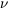e; u, d). The second and third generations consist of (µ-,µ;c, s) and (-,; t, b) respectively. [CD99] (b) Leptons and quarks come two by two. Two leptons (such as electron and neutrino) and two quarks (such as up and down) form a generation. The first generation is (e-,e; u, d), the second is (µ-,µ; c, s) and the third (-, v; t, b). The top quark (t) in the third generation is predicted by some theories but not yet seen. [D89] (c) The fundamental particles of the standard model of particle physics can be grouped into quarks, leptons, force carriers, and the Higgs particle. The quarks and leptons have been found to exist in three generations, each of which is a copy of the first, except that the masses increase with each generation. See Table 7.1 on page 120. (The first generation includes all the building blocks of ordinary matter-electrons and the up and down quarks that make up protons and neutrons. The particles of the other generations were discovered by studying high energy collisions of first generation particles.) [G97] Geocentric (a) Having the Earth at the centre. [A84] (b) With reference to, or pertaining to, the center of the Earth. [S92] Geocentric Coordinates The latitude and longitude of a point on the Earth's surface relative to the center of the Earth; also celestial coordinates given with respect to the center of the Earth. (See zenith; latitude, terrestrial; longitude, terrestrial.) [S92] Geocentric Cosmology (a) School of ancient theories that depicted the earth as standing, immobile, at the center of the universe. [F88] (b) A model of the universe in which the earth is centrally located, and the Sun, planets, and stars revolve around the Earth. [Silk90] Geocorona The outermost part of Earth's atmosphere, a hydrogen halo extending out to perhaps 15 Earth radii, which emits Lyman-radiation when it is bombarded by sunlight. [H76] Geodesic a path or line of shortest distance joining two points in space (or space-time). A geodesic is a straight line if the space is flat. Another familiar geodesic is a great circle on the surface of a sphere. In the general theory of relativity, freely falling particles follow geodesic paths in space-time. [D89] Geodesy Measurement and study of the Earth's size and shape. [A84] Geodetic Coordinates The latitude and longitude of a point on the Earth's surface determined from the geodetic vertical (normal to the specified spheroid). (See zenith; latitude, terrestrial; longitude, terrestrial.) [S92] Geodetic Precession A small, relativistic, direct motion of the equinox along the ecliptic, amounting to 1".915 per century. [H76] Geoid (a) The equipotential surface ("mean sea level") of Earth's gravitational field. [H76] (b) An equipotential surface that coincides with mean sea level in the open ocean. On land it is the level surface that would be assumed by water in an imaginary network of frictionless channels connected to the ocean. [S92] Geology Scientific study of the dynamics and history of the earth, as evidenced in its rocks, chemicals, and fossils. [F88] Geometric Albedo Ratio of the flux received from a planet to that expected from a perfectly reflecting Lambert disk of the same size at the same distance at zero phase angle (cf. Bond albedo). [H76] Geometric Position The geocentric position of an object on the celestial sphere referred to the true equator and equinox, but without the displacement due to planetary aberration. (See apparent place; mean place; aberration, planetary.) [S92] Geometrodynamics (a) A theory which attempts to attribute all physical phenomena to properties of spacetime. (word coined by John Wheeler) [H76] (b) The study of how gravity alters the geometrical properties of space, distorting those properties from the familiar properties of Euclidean geometry. Einstein's theory of gravity, general relativity, is a theory of geometrodynamics. [LB90] Geometry The mathematics of lines drawn through space. In euclidean geometry space is postulated to be "flat", i.e., to be the three-dimensional analog of a plane. In noneuclidean geometry space is "curved", i.e., is the three-dimensional analog of a sphere or a hyperbola. [F88] GermaniumA hard brittle gray metalloid element. Most germanium is recovered during zinc or copper refining as a by-product. Germanium was extensively used in early semiconductor devices but has now been largely superseded by silicon. It is used as an alloying agent, catalyst, phosphor, and in infrared equipment. Symbol: Ge; m.p. 937.45°C; b.p. 2830°C; r.d. 5.323 (20°C); p.n. 32; r.a.m. 72.61. [DC99] Getter A chemical absorption method of removing (pumping) gas from a chamber by tying up molecules on a surface. Activated charcoal. Molecular sieve. See zeolite. [McL97] GeV (a) One thousand million electron-volts. Sometimes called BeV: B for billion (US variety). [D89] (b) One billion (109) electron volts. Sometimes written as one BeV. [F88] (c) A unit of energy, or of mass. A proton weighs about 10-24 grams, which is equivalent to about 1 GeV. One GeV equals 1 billion electron volts. (See electron volt.) [LB90] GFF Giga French Francs. [LLM96] Ghost A faint image near the image required, caused by radiation that has taken a different path. In the case of traditional `silvered' glass mirrors, the main images are caused by light from the backing of the glass. Ghost images, formed by reflection from the front surface of the glass, may be a tenth as bright as the main images. Other `ghost' images may arise following reflections inside the glass. Ghost effects can also arise with non-visible electromagnetic radiations. Thus, ghost television images can appear on the screen if some radio waves reach the antenna after reflection by large nearby objects. This effect can be used to obtain a fairly accurate value for the speed of radio waves.[DC99] GHz Giga Hertz. [LLM96] Giant 1. A star that has evolved off the main sequence and is roughly a hundred times as luminous as the Sun. Giants can be of any color, but yellow, orange, and red giants are the most common. 2. A planet much more massive than Earth. The solar system has four giant planets, all far from the Sun: Jupiter, Saturn, Uranus, and Neptune. [C95] Giant Branch A conspicuous sequence of red stars with large radii in the H-R diagram of a typical globular cluster that extends from the main-sequence turnoff point upward and redward to the red-giant tip. [H76] Giant Molecular Cloud A huge complex of interstellar gas and dust, consisting mostly of molecular hydrogen, that typically stretches over 150 light-years and contains 200,000 solar masses. Giant molecular clouds give birth to new stars. [C95] Giant Stars High-luminosity stars that lie above the main sequence on the Hertzsprung-Russell diagram. [F88] Gibbous An adjective applied to the Moon or Venus when it is more than half full (but not full). [H76] Giga- A prefix meaning 109. [H76] GIS Gas Imaging Spectrometer (ASCA X-ray satellite). Glitch A term used in rocketry to describe a malfunction (or "slide") of the stylus on a chart recorder; also, a sudden change in frequency, as in a pulsar. (Yiddish) [H76] Global Inertial Frame A coordinate system or frame of reference anchored with respect to the overall distribution of matter in the universe. [Silk90] Globular Cluster(a) Spherical, densely populated cluster of older stars. There are a number of such clusters round the edge of our Galaxy. [A84] (b) A star cluster that packs hundreds of thousands of stars into a region only about a hundred light-years across. Bright globular clusters include Omega Centauri, 47 Tucanae, and M13. In the Milky Way, all globular clusters are old. Most are members of the Galactic halo, and the rest are members of the thick disk. [C95] (c) A gravitationally bound spheroidal system of old stars containing about 106 stars. These are amongst the oldest stellar systems known within our own Galaxy and they have very low abundances of the heavy elements relative to the average cosmic abundances. The globular cluster population forms part of the halo population of our Galaxy and it is presumed that these clusters formed early in the formation and evolution of our Galaxy. [D89] (d) A tightly packed, symmetrical group (mass range 104 - 106 M) of thousands of very old (pure Population II) stars. The stellar density is so great in the center that the nucleus is usually unresolved. Their spectra indicate low abundances of heavy elements. Globular clusters are probably the oldest stellar formation in the Galaxy. They are generally found in the halo and are "high-velocity" objects with very elongated orbits around the galactic center. [H76] (e) A spherical congregation of stars within a galaxy that orbit each other because of their mutual gravity. A typical globular cluster has about a million stars; thus globular clusters are much smaller than galaxies. There are about 100 globular clusters in the Milky Way. [LB90] Globule A dense, spherical cloud of dust that absorbs radiation (see Bok globule). [H76] Glueball (a) Hypothesized form of matter consisting entirely of gluons. [D89] (b) Theoretical particles made exclusively of gluons. Tentative evidence of the existence of glueballs had been found in accelerator experiments by the mid-1980s. [F88] Gluon (a) Carrier of interquark force. Plays a role in QCD analogous to that played by the photon in QED. [D89] (b) The hypothetical particle that carries the force between quarks. [P88] (c) Smallest bundle of the strong force field; messenger particle of the strong force. [G99] (d) Gluons are the massless gauge bosons of QCD which mediate the strong color force between quarks. Because of the non-Abelian structure of the theory, gluons can interact with themselves, and may form particles consisting of gluons bound together. The existence of these "glueballs" has yet to be confirmed. [CD99] (e) Quanta that carry the strong nuclear force. Like photons, vector bosons, and gravitons - the carriers respectively of electromagnetism, the weak force, and gravitation - gluons are massless bosons. Consequently, for simplicity's sake, some physicists lump together all the force-carrying quanta under the term "gluons". [F88] (f) The force-carrying particles associated with the strong interactions, the forces which bind quarks inside of protons and neutrons. For more details, see Yang-Mills Theories. [G97] Gluon Lattice Force field generated by the strong nuclear force that holds quarks together. See gluons. [F88] Gödel's Theorem A theorem discovered and proved by the mathematician Kurt Gödel in 1931. Gödel's theorem says that there are certain propositions in each branch of mathematics (such as arithmetic or geometry) whose truth or falsity cannot be proven using methods and results only from that branch of mathematics. [LB90] Golay Cell A gas bulb used to detect infrared radiation. [H76] Gold(a) Element with atomic number 79. It is produced entirely by the r-process, in supernovae. [C95] (b) A transition metal that occurs native. It is unreactive and is very ductile and malleable. Gold is used in jewelry, often alloyed with copper, and in electronics and colored glass. Pure gold is 24 carat; 9 carat indicates that 9 parts in 24 consist of gold. Symbol: Au; m.p. 1064.43°C; b.p. 2807°C; r.d. 19.320 (20°C); p.n. 79; r.a.m. 196.96654. [DC99] Goldstone Boson A massless spin-0 particle which arises whenever a (continuous) global symmetry is spontaneously broken. [CD99] Gould Belt The local system of stars and gas within about 300 pc of the Sun. It is a belt inclined about 10°-20° to the galactic plane in which the greatest concentration of naked-eye O and B stars occurs. [H76] GPS Global Positioning System. [LLM96] GPS Radio Source Gigahertz Peaked Spectrum A class of powerful compact extragalactic radio sources characterized by an overall turnover in their radio spectra at about 1 GHz in frequency. Graceful Exit Problems A problem of the original formulation of the inflationary theory, in which the formation of bubbles at the end of inflation destroys the homogeneity of the universe. See decay of the false vacuum, and percolation. [G97] Grand Unification (a) An attempt to produce a unification of all the forces of nature. While some success was made in unifying the gluon force between quarks with the electroweak force, problems always arose when gravity was included. Grand unification eventually gave way to superstring theory. [P88] (b) Class of theories that merge all three nongravitational forces into a single theoretical framework. [G99] Grand Unified Theory (a) A mathematical scheme in which the electromagnetic, weak and strong nuclear forces are unified into a consistent description. [D89] (b) Class of theories that purport to reveal identities linking the strong and electroweak forces. The differences between these forces in nature today is attributed to the breaking of symmetrical relationships among force-carrying particles as the very early universe expanded and cooled. [F88] (c) A speculative class of theories of particle interactions, first developed in 1974, which attempt to describe electromagnetism, the weak interactions, and the strong interactions in a fully unified theory. Of the known forces, only gravitation is omitted. [G97] (d) Theories in physics that attempt to explain the forces of nature as manifestations of a single underlying force. [LB90] Granulation The mottled appearance of the solar photosphere, caused by gases rising from the interior of the Sun (see granules). [H76] Granules Convective cells (about 1000 km in diameter) in the solar photosphere. Each granule lasts about 5 minutes on the average and represents a temperature roughly 300° higher than the surrounding dark areas. At any one time, granules cover about one-third of the solar photosphere. [H76] Graticule A transparent plate or cell bearing a grid, cross-wire, or scale, designed to be used with an optical instrument or cathode-ray oscilloscope for the purpose of positioning, measuring, or counting objects in the field of view; the scale, grid, etc., on such a plate. Grating See diffraction grating. [A84] Gravitation (a) The universal ability of all material objects to attract each other; F = Gm1m2 / r2. [H76] (b) The mutual attraction between any two masses, as was first described accurately by Newton. Gravity appears strong because it has infinite range and it is always attractive (except for a false vacuum), but on a subatomic level gravity is the weakest of the known interactions; the gravitational force between a proton and an electron is 2 × 1039 times weaker than the electrical attraction. [G97] Gravitational Collapse The sudden collapse of a massive star when the radiation pressure outward is no longer sufficient to balance the gravitational pressure inward. In gravitational collapse there is a sudden, catastrophic release of great quantities of gravitational potential energy, and this release has been postulated as the cause of supernovae, neutron stars, and black holes. [H76] Gravitational Constant (a) Fundamental constant with units of cm3 g-1 s-2 that determines the gravitational force between two bodies at a given separation. [D89] (b) The constant of proportionality in the attraction between two unit masses a unit distance apart. G = 6.668 × 10-8 dyn cm2 g-2. [H76] (c) A physical constant of proportionality which occurs in the equation giving the force of attraction existing between any two material bodies. Thus if the two bodies are of masses m1 and m2 respectively and are at a distance r apart, the force of attraction F is given by the equation F = G × m1 × m2/r2, where G is the gravitational constant. No direct connection has, as yet, been established between G and any other physical quantities and increases in the accuracy of its measurement have made no difference to the values of the other physical constants. One of the earliest determinations of G was made by Henry Cavendish in 1798 when, by using a torsion balance method, he obtained a value of 66.74 × 10-12 m3 kg-1 s-2; this differs but little from the modern accepted value of 66.7259 × 10-12 m3 kg-1 s-2 obtained by Luther and Towler in 1982. A list of all the papers published on the determination of G has recently been compiled by T. G. Gillies. [JM92] (d) A fundamental constant of nature that measures the strength of the gravitational force. The gravitational constant is also called Newton's gravitational constant and is denoted by G. [LB90] Gravitational Encounter The encounter between two massive bodies which results in the deviation from their original directions of motion. [H76] Gravitational Energy Same as gravitational potential energy. [G97] Gravitational Equilibrium The condition in a star in which at each point the weight of the overlying layers is balanced by the total pressure. [H76] gravitational field Instead of describing gravity as an action-at-a-distance force, modern physicists describe it in terms of a gravitational field. At each point of space, the field is defined as the force that would be experienced by a standard mass, if the mass were positioned at that point. While Newton's law of gravity can be expressed equally well in terms of an action-at-a-distance or a field, Einstein's theory of general relativity, which is now the accepted description of gravity, can be formulated only in terms of fields. [G97] Gravitational Field Lines A method of depicting a gravitational field by drawing lines. The direction of the field is indicated by the direction of the lines, and the strength of the field is indicated by how closely the lines are spaced. [G97] Gravitational Force (a) The weakest of the four fundamental forces of nature, the gravitational force between any two masses is proportional to the product of the masses and varies inversely as the square of the distance between them. The other three fundamental forces are the electromagnetic force and two kinds of nuclear forces. (See electromagnetic force; nuclear forces.) [LB90] (b) The weakest of the four fundamental forces of nature. Described by Newton's universal theory of gravity, and subsequently by Einstein's general relativity. [G99] (c) As described first by Isaac Newton, gravity is a force that exists between bodies of any mass whatever (from particles to stars) in proportion to the product of their masses, and in inverse proportion to the square of the distance between them. The weakest of the four natural forces (the other three being the electromagnetic and the two nuclear interactive forces), its real nature is still not fully understood. Einstein's General Theory of Relativity presented another viewpoint. [A84] (d) Fundamental force of nature, generated by all particles that possess mass. Interpreted by means of Newtonian mechanics or by the general theory of relativity. [F88] Gravitational Instability The process by which fluctuations in an infinite medium of size greater than a certain length scale (the Jeans length) grow by self-gravitation. In the expanding universe, the growth is slowed by the expansion, but the unstable fluctuations eventually collapse into stable, gravitationally bound systems. [Silk90] Gravitational Lens (a) A galaxy that intervenes between us and a distant astronomical object and that gravitationally deflects the light from that distant object. (Light, like matter, is attracted by gravity.) Gravitational lenses can focus, distort, and split light beams in the same way that ordinary glass lenses do. [LB90] (b) Deflection of electromagnetic radiation from a distant background source by a strong gravitational field associated with a foreground source resulting in more than one image of the original source. Many double-quasars are produced by this phenomenon. [McL97] Gravitational-Lens Effect The effect of matter in curved spacetime, which tends to focus any beam of radiation from a distant source. In effect, the spacetime curvature is a lens of great focal length. At z1, the angular size of an object starts increasing with distance. [H76] Gravitational Lensing The bending of light caused by the gravity of an object lying between us and the light source. This may cause the light source to look brighter than it normally does. [C95] Gravitational Mass That property of matter which makes it create a gravitational field and attract other particles (cf. inertial mass; equivalence principle). [H76] Gravitational Potential Energy (a) Energy that a body can acquire by falling through a gravitational field and that decreases as the kinetic energy increases. There is no general reference level (analogous to the state of rest of a body in defining kinetic energy), and so we customarily define the change in gravitational potential energy as the negative of the work done by the gravitational forces during the bodys change of position. [Silk90] (b) When we lift a weight from the floor to a tabletop, we clearly put energy into it. The energy is not lost, however, because we can retrieve it by allowing the weight to fall back to the floor. While the weight is on the table, we say that the energy is stored as gravitational potential energy. The energy is stored in the gravitational field. [G97] Gravitational Radiation (a) Propagating waves of gravitational tidal force that are emitted by dynamical systems such as collapsing stars or binary star systems, and move with the speed of light. [D89] (b) According to general relativity, any massive body with variable quadrupole and higher moments must emit gravitational waves. In the lowest approximation, the radiation emitted is derived from the varying quadrupole moment of the object; more generally, any body which experiences varying acceleration will emit gravitational waves by an amount proportional to the rate of change of the acceleration. [H76] Gravitational Radius The radius which an object should have in order that light emitted from its surface just ceases to escape from its surface. [H76] Gravitational Redshift (a) Generic name for the shift in the frequency or wavelength of a signal that travels up or down in a gravitational field; effect is a redshift if signal travels upward, a blueshift if it travels downward. [D89] (b) The rate at which a clock keeps time when it is in a gravitational field is slower than the rate at which it will keep time in the absence of a gravitational field. (The gravitational redshift was experimentally verified by Pound and Rebka in 1960.) The amount of redshift is directly proportional to the mass of the emitting body and inversely proportional to its radius. [H76] (c) Light is emitted at a lower frequency and longer (or redder) wavelength in a gravitational field than in the absence of a gravitational field. The gravitational redshift of the radiation from a white dwarf star amounts to about 1 part in 104; this effect has also been measured for the earth's gravitational field, where it amounts to 1 part in 109. Near a black hole, the effect can be much larger, being proportional to the mass of the body divided by its radius. [Silk90] Gravitational Sigularity a region where the gravitational field has become so strong that the curvature of space-time is infinite. The occurrence of such a situation signals the breakdown of the theory and is a central feature of the classical theory of relativity. [D89] Gravitational TheoriesGravitational Waves Disturbances or ripples in spacetime predicted by the General Theory of Relativity due to changing distributions of mass such as the spindown of a neutron star binary system or the implosion of a star during a supernova. No confirmed direct detections so far. [McL97] Gravitino (a) The fermion partner of the graviton predicted by the supergravity extension of Einstein's theory of general relativity. [D89] (b) Hypothetical force-carrying particles predicted by supersymmetry theories. The gravitino's spin would be 1/2. Its mass is unknown. [F88] Graviton (a) A massless spin-2 particle which is the hypothetical quantum of the gravitational field. It mediates the force of gravity in a similar way to that in which the spin-1 gauge bosons (i.e. the photon, W±, Z0, and gluons) mediate the other forces. [CD99] (b) The quantum of the gravitational field. In the quantum theory of general relativity, this elementary particle is massless and has a spin value of two. [D89] (c) A hypothetical elementary particle associated with the gravitational interaction. It is a stable particle with zero rest mass, zero charge, and a spin of ± 2, and travels with the speed of light. [H76] (d) Smallest bundle of the gravitational force field; messenger particle for the gravitational force. [G99] (e) The hypothetical quantum particle of the gravitational field. It could also be thought of as a quantized element of space-time curvature. [P88] (f) A quantum of gravitational radiation. [Silk90] (g) The quantum thought to convey gravitational force; analogous to the photons, gluons, and intermediate vector bosons of electromagnetism and the strong and weak nuclear forces. Predicted by quantum theory of gravity, gravitons have not yet been detected. [F88] Gravity (a) One of the four fundamental forces of nature, and the one most different from the other three. [C97] (b) In Aristotelian physics, an innate tendency of the elements earth and water to fall. (c) In Newtonian physics, the universal, mutual, attraction of all massive objects for one another; its force is directly proportional to the mass of each object, and decreases by the square of the distance separating the objects involved. (3) In Einstein's general relativity, gravity is viewed as a consequence of the curvature of space induced by the presence of a massive object. In quantum mechanics the gravitational field is said to be conveyed by quanta called gravitons. [F88] Gravity Darkening See von Zeipel's theorem. [H76] Gravity Waves Traveling waves of energy that transmit a gravitational force whose strength is changing in time. Gravitational waves are analogous to the electromagnetic waves that transmit the electromagnetic force. [LB90] Gray Atmosphere A model atmosphere in which the continuous absorption coefficient is assumed to be independent of frequency. [H76] Gray Body A body whose emissivity is constant and less than unity. [H76] Grazing-Incidence Telescope A telescope used in X-ray and gamma-ray astronomy. It focuses these rays by making use of the fact that they behave like light rays if they strike surfaces at a shallow enough angle. [H76] Great Attractor A hypothesized large mass, some hundred million light years from earth, that seems to be affecting the motions of many nearby galaxies by virtue of its gravity. [LB90] Great Looped Nebula See 30 Doradus. [H76] Great Red Spot See Red Spot. [H76] Great Rift A "split" in the Milky Way between Cygnus and Sagittarius caused by a succession of large, overlapping dark clouds in the equatorial plane of the Galaxy. It is about 100 pc distant. [H76] Great Wall A sheet of galaxies which stretches more than 500 million light-years across the sky. [G97] Great Year Ancient concept of a celestial and historical cycle, its duration roughly a thousand or ten thousand years, at the end of which there is universal destruction and a new year begins. [F88] Green's Theorem An identity that connects line integrals and double integrals. [H76] Greenhouse Effect Retention and escalation of temperature beneath a mantle of clouds or denser atmosphere. [A84] Greenwich Site now in London of the first Royal Greenwich Observatory, designed and built by Christopher Wren in 1675. [A84] Greenwich Sidereal Date (GSD) The number of sidereal days elapsed at Greenwich since the beginning of the Greenwich sidereal day that was in progress at Julian date 0.0. [S92] Greenwich Sidereal Day Number The integral part of the Greenwich sidereal date. [S92] Gregorian A class of reflecting telescope which uses a concave secondary mirror placed after the prime focus is reached instead of a convex secondary placed before the prime focus. [McL97] Gregorian Calendar (a) Calendar established with the authority of the Roman Catholic Church by Pope Gregory XIII in 1582. Correcting at a stroke the 10-day accumulated margin of error of the Julian calendar, the main difference was in fact that century years were discounted as leap years unless they were divisible by 400. [A84] (b) The calendar introduced by Pope Gregory XIII in 1582 to replace the Julian calendar; the calendar now used as the civil calendar in most countries. Every year that is exactly divisible by four is a leap year, except for centurial years, which must be exactly divisible by 400 to be leap years. Thus 2000 is a leap year, but 1900 and 2100 are not leap years. [S92] Gregorian Telescope (a) Telescope devised - but never constructed - by James Gregory, in which an auxiliary concave mirror reflects the magnified image, the right way up, through a hole in the centre of the main objective mirror, i.e., through the end of the telescope itself. The Cassegrain telescope is similar but produces an inverted image. [A84] (b) An early type of reflecting telescope similar to the Cassegrainian arrangement, except that both mirrors were converging. See also reflector. [DC99] GRG Giant Radio Galaxies, represent the biggest single objects in the Universe, having linear sizes larger than 1 Mpc. GRGs are rare among the entire population of radio galaxies, and their physical evolution is not well understood. GRGs are of special interest for several reasons: The lobes of radio sources can compress cold gas clumps and trigger star or even dwarf galaxy formation, they can also transport gas from a host galaxy to large distances and seed the IGM with magnetic fields. Since GRGs are larger in size than normal radio galaxies by anywhere from 10 to 100 times, their influence on the ambient medium is correspondingly wider and is pronounced on scales comparable to those of clusters of galaxies or larger. GRGs could therefore play an important role in the process of large-scale structure formation in the Universe. [BFM2004] Grism This is a right-angled glass prism with a transmission diffraction grating deposited on the hypotenuse surface. The spectrum produced by the grating is deflected by the prism to remain on the optical axis and the apex angle of the prism is chosen to get a certain wavelength in the center of the detector. Grisms can be placed in a filter wheel. [McL97] Groombridge 1830 A famous halo star that lies 28 light-years away in the constellation Ursa Major. Its proper motion, discovered in 1841, was then the largest known, displacing that of 61 Cygni. [C95] Grotrian Diagram Energy-level diagram. [H76] LEVEL5: Grotrian Diagrams Ground State (a) The lowest energy state of an atom, molecule, or other system. [DC99] (b) The state in which all electrons are in the lowest possible energy states. [H76] Ground Loop A condition in which two pieces of apparatus are connected together while each has a separate earth connection and these are not at identical potentials. A current will flow and small signals will be affected by fluctuations, called noise. [McL97] Group A small gathering of galaxies, smaller than a cluster. The Milky Way is part of the Local Group, which contains about thirty galaxies. [C95] Group Theory The branch of mathematics which describes symmetry. A mathematical group G is defined as a collection of elements {a, b, c,....} with the properties: (1) if a and b are in group G, then the product of the two elements, ab, is also in G (2) there is a unit element e such that ae = a for all elements a in G; (3) each element a has an inverse a-1 such that aa-1 = e. So, for instance, the rotations of an (x, y) coordinate system about the z-axis form a group, since the effect of two rotations12 is equivalent to the effect of one big rotation3. Such a group is called a continuous group as the angles of rotation can vary continuously. In general, the elements of a group may be represented by matrices, which form various "representations" of the group. These representations may be used to determine how a physical system changes under the action of symmetry transformations. Moreover, when a system possesses a symmetry which is described by a group G (i.e. when its equations of motion are left invariant under group transformations), then the various representations specify the symmetry properties of the relevant degrees of freedom. For example, hadrons appear to possess an SU(3) "flavor" symmetry. The fundamental 3-dimensional representation of SU(3) contains the three flavor degrees of freedom associated with the up, down and strange quarks: 3 = (u, d, s). The eight-dimensional representation 8 of SU(3) contains the eight flavor degrees of freedom associated with the meson and baryon octets. Furthermore, a given representation completely specifies the flavor quantum numbers of associated particles. The same is true for local gauge (i.e. dynamical) symmetries. For example, the theory of QCD possesses a local SU(3)C color symmetry. The three-dimensional representation of SU(3)c contains the three color degrees of freedom associated with the color charges red, green and blue: 3 = (r, g, b). The eight-dimensional representation contains the eight color degrees of freedom associated with the eight gluons the gauge bosons of QCD. Discrete groups having a finite number of elements are associated with discrete symmetry transformations, such as parity. For example, the discrete group corresponding to parity has only two elements: P and P2 = e. GSD Greenwich Sidereal Date The number of sidereal days elapsed at Greenwich since the beginning of the Greenwich sidereal day that was in progress at Julian date 0.0. [S92] GSFC G-Type Star A star having a spectral type of G, that is, yellow, like the Sun. [C95] GUI Graphical user interface. [McL97] Guillotine Factor A factor that measures the sharp reduction in the opacity of a gas when the temperature is high enough to have ionized the atoms down to their K shells. [H76] Gum Nebula A giant H II region 30°-40° in diameter in which the Vela pulsar and the Vela X supernova remnant are embedded. It appears to be a fossil Strömgren sphere produced by an outburst of ionizing radiation that accompanied the Vela X supernova remnant. (Named for the Australian astronomer Colin Gum.) [H76] GUT Grand Unified Theory. [F88] Gyrofrequency The frequency with which an electron or other charged particle executes spiral gyrations in moving across a magnetic field. [H76] Gyrosynchrotron Radiation Radiation emitted by mildly relativistic electrons. [H76]